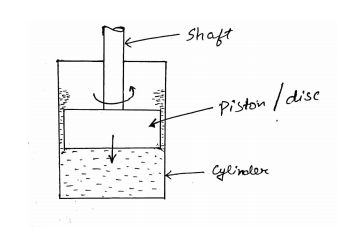# Damped free Vibration - Numerical 2

### The disc of a torsional pendulum has a moment of inertia of 600 $kg \sdot cm^2$ and is immersed in viscous fluid. The brass shaft attached to it is of $10 \ cm$ diameter and $40 \ cm$ long. When the pendulum is vibrating the observed amplitudes on the same side of the rest position for successive cycle are $9 \degree$, $6 \degree$, $4 \degree$. Determine the following:

1. Logarithmic Decrement
2. Damping torque at unit velocity
3. The periodic time of vibration

Assume for the brass shaft, $G = 4.4⨉10^{10} N/m^2$. What would be the frequency if the disc is removed from the viscous fluid?

### Solution

Given data:

\begin{aligned} \text{Moment of inertia of disc,} \ \ I &= 600 \ kg \sdot cm^2 \\ &= 600 \times 10^{-4} \ kg \sdot m^2 \\ &= 0.06 \ kg \sdot m^2 \\ \\ \text{Diameter of the shaft,} \ \ d &= 10 \ cm = 0.1 \ m \\ \\ \text{Length of the shaft,} \ \ L &= 40 \ cm = 0.4 \ m \\ \\ \text{Initial amplitude,} \ \ x_0 &= 9 \degree \\ \\ \text{Second amplitude,} \ \ x_1 &= 6 \degree \\ \\ \text{Third amplitude,} \ \ x_2 &= 4 \degree \\ \end{aligned}

## 1. The logarithmic decrement:

\begin{aligned} \delta &= {\log}_e \bigg( {x_0 \over x_1}\bigg) \\ \\ &= {\log}_e \bigg( {9 \over 6}\bigg) \\ \\ &= {\log}_e (1.5) \\ \\ &= 0.405 \end{aligned}## 2. Damping torque at unit velocity:

• The damping factor is,
\begin{aligned} \xi &= {\delta \over \sqrt{4 {\pi}^2 + {\delta}^2}} \\ &= {0.405 \over \sqrt{4 {\pi}^2 + (0.405)^2}} \\ &= 0.0642 \end{aligned}
• The torsional stiffness of shaft is,
\begin{aligned} K_t &= {GJ \over L} \\ \\ &= {G \sdot {\pi \over 32} \sdot (d)^4 \over L} \\ \\ &= {4.4 \times 10^{10} \times {\pi \over 32} \sdot (0.1)^4 \over 0.4} \\ \\ &= 1.07 \times 10^6 \ Nm/rad \end{aligned}• The natural circular frequency of system is,
\begin{aligned} {\omega}_n &= \sqrt{K_t \over I} \\ \\ &= \sqrt{1.07 \times 10^6 \over 0.06} \\ \\ &= 4242.5 \ \ rad/s \end{aligned}

Now, Damping torque,

$F_t = c_t \sdot \mathring{x}$

$\therefore$ Damping torque at unit velocity, ${F_t \over \mathring{x}} = c_t$

Now, we know that;

$\xi = {c_t \over c_c}$ \begin{aligned} \therefore c_t &= \xi \sdot c_c \\ &= \xi (2 \sdot I \sdot {\omega}_n) \\ &= 0.0642(2 \times 0.06 \times 4242.5) \\ &= 32.74 \ \ N \sdot m \sdot s / rad \end{aligned}

## 3. The time period of damped vibration:

\begin{aligned} t_p &= {2\pi \over {\omega}_d} \\ \\ &= {2\pi \over {\omega}_n \sqrt{1 - {\xi}^2}} \\ \\ &= {2\pi \over (4242.5) \sqrt{1 - (0.0642)^2}} \\ \\ &= 0.00148 \ \ second \end{aligned}

## 4. Frequency, if disc is removed from viscous fluid:

\begin{aligned} f_n &= {{\omega}_n \over 2\pi} \\ \\ &= {4242.5 \over 2\pi} \\ \\ &= 675.21 \ Hz \end{aligned}